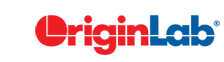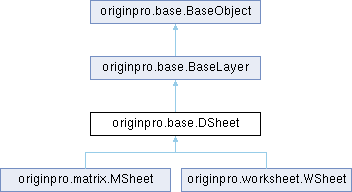OriginPro
Searching...
No Matches
originpro.base.DSheet Class Reference
Inheritance diagram for originpro.base.DSheet:## Public Member Functions

def __str__ (self)

def __repr__ (self)

def shape (self)

def shape (self, val)

def remove_DC (self)

def has_DC (self)

def from_file (self, fname, keep_DC=True, dctype='', sel='', sparks=False)

def lt_range (self, use_name=True)

def get_book (self)

def get_labels (self, type_='L')

def set_labels (self, labels, type_='L', offset=0)

def tabcolor (self)

def tabcolor (self, rgb)Public Member Functions inherited from originpro.base.BaseLayer
def __str__ (self)

def activate (self)

def destroy (self)Public Member Functions inherited from originpro.base.BaseObject
def __init__ (self, obj)

def __del__ (self)

def __str__ (self)

def __bool__ (self)

def index (self)

def get_str (self, prop)

def get_int (self, prop)

def get_float (self, prop)

def set_str (self, prop, value)

def set_int (self, prop, value)

def set_float (self, prop, value)

def method_int (self, name, arg='')

def method_float (self, name, arg='')

def method_str (self, name, arg='')

def lt_exec (self, labtalk)

def name (self)

def name (self, value)

def lname (self)

def lname (self, value)

def show (self)

def show (self, value)

def usertree (self)

def usertree (self, tr)

def userprops (self)Public Attributes inherited from originpro.base.BaseObject
obj

## Detailed Description

`base class for data sheets, like worksheets and matrix sheets`

## ◆ __str__()

 def originpro.base.DSheet.__str__ ( self )

Reimplemented from originpro.base.BaseLayer.

## ◆ from_file()

 def originpro.base.DSheet.from_file ( self, fname, keep_DC = `True`, dctype = `''`, sel = `''`, sparks = `False` )
```Imports data from a file using a Data Connector.

Parameters:
fname (str): File path and name to import.
keep_DC (bool): Keep the Data Connector in the book after import
dctype (str): Data Connector name, like "Import Filter", "MATLAB", "NetCDF", if not specified, CSV or Excel connector will be used based on file name
sel (str): selection in the file, this will depend on the connector
sparks (bool): Allows sparklines or not, True will follow GUI setting to add sparklines, False will disable it completely
Returns:
None

Examples:
wks=op.find_sheet()
fn=op.path('e') + 'Samples\\Import and Export\donations.csv'
wks.from_file(fn, False)#remove connector after import to allow further edit of data
wks2=op.new_sheet()
wks2.from_file(op.path()+'test.xlsx')#assuming you have this in UFF(user files folder)
```

## ◆ get_book()

 def originpro.base.DSheet.get_book ( self )

## ◆ get_labels()

 def originpro.base.DSheet.get_labels ( self, type_ = `'L'` )

## ◆ has_DC()

 def originpro.base.DSheet.has_DC ( self )
```Returns whether a sheet has a Data Connector.

Parameters:

Returns:
(str) name of the Data Connector, like 'csv', 'excel'

Examples:
dc = wb.has_DC()
if len(dc):
print(f'sheet is connected using {dc}')
```

## ◆ lt_range()

 def originpro.base.DSheet.lt_range ( self, use_name = `True` )
```Parameters:
use_name(bool):
Returns:
Return the Origin Range String that identify Data Sheet object
Examples:
ws=op.find_sheet()
print(ws.lt_range())```

## ◆ remove_DC()

 def originpro.base.DSheet.remove_DC ( self )
```Removes Data Connector from a sheet.

Parameters:

Returns:
None

Examples:
wks.remove_DC()
```

## ◆ set_labels()

 def originpro.base.DSheet.set_labels ( self, labels, type_ = `'L'`, offset = `0` )

## ◆ shape() [1/2]

 def originpro.base.DSheet.shape ( self )
```Parameters:
none
Returns:
(tuple) return the rows and columns of a sheet
Examples:
wks=op.find_sheet()
print(wks.shape)
```

## ◆ shape() [2/2]

 def originpro.base.DSheet.shape ( self, val )
```setting the number of rows and columns in a sheet
Parameters:
val (tuple): rows, cols, 0 = unchanged
Returns:
(tuple) the new shape
Examples:
wks=op.find_sheet()
#leave rows unchanged and set to 3 columns
wks.shape=0,3
```

## ◆ tabcolor() [1/2]

 def originpro.base.DSheet.tabcolor ( self )
```Parameters:
none
Returns:
returns the LabTalk color code of the sheet tab
If sheet tab has no custom color 0 will be returned
Examples:
cc = wks.tabcolor
if cc:
r, g, b = op.to_rgb(cc)
```

## ◆ tabcolor() [2/2]

 def originpro.base.DSheet.tabcolor ( self, rgb )
```Property setter for the sheet tab color, use 0 to clear

Parameters:
rgb(int, str, tuple): various way to specify color, see function ocolor(rgb) in op.utils

Returns:
None

Examples:
wks.tabcolor = 'Red'
wks.tabcolor = '#00f'
wks.tabcolor = 0
```

The documentation for this class was generated from the following file:
• src/originpro/base.py